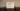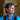# LINQ for beginners: pick the right methods!LINQ is one of the most loved functionalities by C# developers. It allows you to perform calculations and projections over a collection of items, making your code easy to build and, even more, easy to understand.

As of C# 11, there are tens of methods and overloads you can choose from. Some of them seem similar, but there are some differences that might not be obvious to C# beginners.

In this article, we're gonna learn the differences between couples of methods, so that you can choose the best one that fits your needs.

## First vs FirstOrDefault

Both `First` and `FirstOrDefault` allow you to get the first item of a collection that matches some requisites passed as a parameter, usually with a Lambda expression:

``````int[] numbers = new int[] { -2, 1, 6, 12 };

var mod3OrDefault = numbers.FirstOrDefault(n => n % 3 == 0);
var mod3 = numbers.First(n => n % 3 == 0);``````

Using `FirstOrDefault` you get the first item that matches the condition. If no items are found you'll get the default value for that type. The default value depends on the data type:

Data type Default value
int 0
string null
bool false
object null

To know the default value for a specific type, just run `default(string)`.

So, coming back to `FirstOrDefault`, we have these two possible outcomes:

``````int[] numbers = new int[] { -2,  1, 6, 12 };
numbers.FirstOrDefault(n => n % 3 == 0); // 6
numbers.FirstOrDefault(n => n % 7 == 0); // 0``````

On the other hand, `First` throws an `InvalidOperationException` with the message "Sequence contains no matching element" if no items in the collection match the filter criterion:

``````int[] numbers = new int[] { -2,  1, 6, 12 };
numbers.First(n => n % 3 == 0); // 6
numbers.First(n => n % 7 == 0); // throws InvalidOperationException``````

## First vs Single

While `First` returns the first item that satisfies the condition, even if there are more than two or more, `Single` ensures that no more than one item matches that condition.

If there are two or more items that passing the filter, an `InvalidOperationException` is thrown with the message "Sequence contains more than one matching element".

``````int[] numbers = new int[] { -2, 1, 6, 12 };
numbers.First(n => n % 3 == 0); // 6
numbers.Single(n => n % 3 == 0); // throws exception because both 6 and 12 are accepted values``````

Both methods have their corresponding `-OrDefault` counterpart: `SingleOrDefault` returns the default value if no items are valid.

``````int[] numbers = new int[] { -2, 1, 6, 12 };

numbers.SingleOrDefault(n => n % 4 == 0); // 12
numbers.SingleOrDefault(n => n % 7 == 0); // 0, because no items are %7
numbers.SingleOrDefault(n => n % 3 == 0); // throws exception``````

## Any vs Count

Both `Any` and `Count` give you indications about the presence or absence of items for which the specified predicate returns True.

``````int[] numbers = new int[] { -2, 1, 6, 12 };

numbers.Any(n => n % 3 == 0); // true
numbers.Count(n => n % 3 == 0); // 2``````

the difference is that `Any` returns a boolean, while `Count` returns an integer.

## Where vs First

As you remember, `First` returns only one item.

If you need all the items that meet the specified criteria, you can use `Where`:

``````int[] numbers = new int[] { -2, 1, 6, 12 };
numbers.Where(n => n % 3 == 0); // [6, 12]``````

## Sort vs Order

Both `Sort` and `Order` deal with the sorting of collections.

The main difference is that `Sort` sorts the items in place, modifying the original collection.

On the contrary, `Order` and `OrderBy` create a new collection of items with the same items of the original sequence but sorted.

``````List<int> originalNumbers = new List<int> { -7, 1, 5, -6};
originalNumbers.Sort(); // originalNumbers now is [-7, -6, 1, 5]``````

Also, notice that `Sort` is valid only on `List<T>`, and not Arrays or generic Enumerables.

`OrderBy` and `Order` create a brand-new collection of items.

``````List<int> originalNumbers = new List<int> { -7, 1, 5, -6};
var sortedNumbers = originalNumbers.OrderBy(n => n);
// sortedNumbers is [-7, -6, 1, 5];
// originalNumbers is [-7, 1, 5, -6];``````

💡 Starting from C# 11 we can simplify `OrderBy(n => n)` and use `Order()`!

C# collections do not natively expose such methods. They are ALL Extension methods (well, except for `Sort`, which is a native of `List<T>` ).

If you want to learn what are Extension Methods and how you can write your own methods, have a look at this article:

Then, in the C# TIPS section of my blog, there are several articles that you might find interesting.

One of these is about a LINQ method that you might want to know: `SelectMany`.

If you want to learn more about `Sort`, the best place is the documentation:

## Wrapping up

In this article, we learned the differences between couples of LINQ methods.

Each of them has a purpose, and you should use the right one for each case.

❓ A question for you: talking about performance, which is more efficient: `First` or `Single`? And what about `Count() == 0` vs `Any()`? Drop a message below if you know the answer! 📩